English

MQL4 BookBasics of MQL4Functions

# Functions

## The term of function

The most important technological breakthrough in computer engineering is the possibility of creation and storage of separate code fragments that describe the rules of data processing for solving a problem or completing a small task. Such a possibility exists in MQL4, too.

A function is a named, specific part of a program that describes a method of data conversion.

We will consider two aspects of functions: function descriptions and function calls.

A function description is a specific, named part of a program intended for execution.

A function call (or, function reference) is a record, the execution of which results in the execution of the function to which it refers.

In our everyday life, we can find many analogues of the function. Take, for example, the braking system of a car. The actuating mechanism that properly performs braking, as well as the idea implemented by the engineer, is like a function, whereas the brake pedal is like a function call. The driver presses the pedal down, and the actuating mechanisms perform some actions and stop the car.

Similarly, if a function call occurs in a program, then the function of the same name will be called and executed. That is, a certain sequence of calculations or other actions will be performed, such as displaying a message or opening an order. The general use of a function is to take a logically completed part of the code out of the basic text of the program and to replace it with only the call for this part of the code. Such program construction has some incontestable advantages.

First, the program text composed in such a manner is read much easier.

Second, one can easily see and modify the text of a function without making any changes in the basic code of the program.

Third, a function can be composed as a single file and used in other programs. This releases the programmer from the necessity to insert the same fragments of the code in each newly created program.

Most code in MQL4 is written in form of functions. This approach became widespread and is a standard now.

## Composition of a function

Thus, a function can be described and called. Let us consider an example. Suppose we have a small program, Figure 18, that finds the length of a hypotenuse using the two other sides of the right triangle and the Pythagorean theorem.In this program, all calculations are located together. The operators are executed one at a time in the order in which they occur in the program (from the top down).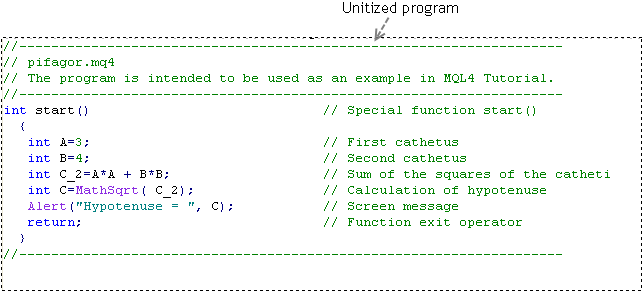Figure 18 The code of a single program pifagor.mq4.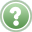Problem 8 Compose a part of the given program code as a function.

It would be reasonable to make a function using the two lines that find the search value. The same program using a function is shown in Figure 19.In this program, part of the calculations is composed as a function. The basic code contains a user-defined function call. The description of the user-defined function is located outside (after) the basic code.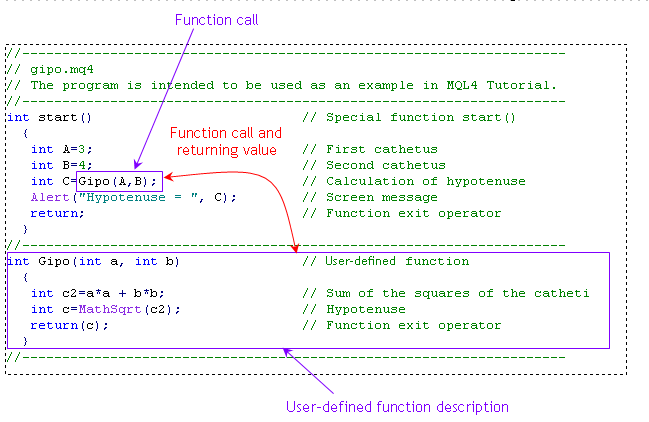Figure 19 The code of a program that contains the description of a user-defined function and the call for it gipo.mq4.

Both versions of the program will give the same result. However, the code is composed as a single module in the first version in Figure 18, whereas in the second version in Figure 19, a part of the calculations is executed in a function that is called from the basic text. Upon completion of the calculations within the function, the calculations in the main code will be continued.

To have the function executed, we have to call it (refer to it). This is why the function is practically represented in two parts: the code itself composing the function (function description) and the function call used to launch the function (referring to the function). If you do not call the function, it will not be executed. At the same time, if you call a nonexistent function, this will result in nothing. Actually, MetaEditior will give an error message if you try to compile such a program in MQL4.

## Function description

The description of a function consists of two basic parts: the function header and the function body.

The header of a function consists of the type of the return value, the function name, and the list of formal parameters. The list of formal parameters are enclosed in parentheses and placed after the function name. The type of the return value can be one of the types we already know: int, double, bool, color, datetime, or string. If the function does not return any value, its type can be denoted as void (without contents, empty) or as any other type.

The body of a function is enclosed in braces. The function body may contain simple operators and/or compound operators, and it may contain calls for other functions. The value returned by the function is given in the parentheses of the return() operator. The type of the value returned using return() must match with the function type specified in the function header. The description of the function is ended with a closing brace.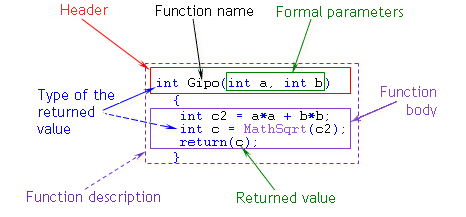Figure 20 Function description.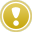The function description must be located separately in the program, outside any other functions (that is, not inside of a function).

## Function call

A function call includes the function name and the list of transferred parameters. The list of transferred parameters is enclosed in parentheses and separated by commas. The function call can be represented as a separate operator or as a part of an operator.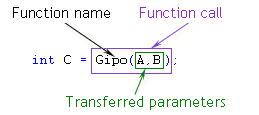Figure 21 Function call (reference to a function).Any function call is always specified within another function (that is, not outside all other functions, but inside one of them).

## Function types

There are three types of functions: special functions, standard (built-in, predefined) functions, and user-defined functions.

### Special functions

In MQL4, there are three special functions in total. They have predefined names: init(), start(), and deinit(). These may not be used as the names of any other functions. A detailed consideration of special functions is given in Special functions. We only say here that the basic code of a program is located inside these functions, as in Figures 18 and 19.

The special feature of special functions is the fact that they are called for execution by the client terminal. Although special functions have all the properties of functions in general, they are not usually called from the program if this program is coded properly.

### Standard functions

MQL4 has a number of useful functions that do not need to be described when coding a program. For example, doing things like computing square roots, printing messages in the system journal, and many other standard functions are executed according to their predefined algorithms. You need not learn the contents of these functions. You can just be sure that all standard functions are developed by professionals properly and according to the best possible algorithm.

The special feature of standard functions is that they are not described in the text of the program. The standard functions are simply called in the program in the same manner as when using any other function. (It is a common practice among coding languages.)

In our example (Figures 18 and 19), two standard functions are used: MathSqrt() and Alert(). The former one is intended for computing square roots, whereas the latter one is for printing a message on the screen. The properties of standard functions are considered in more detail in Standard functions. Here, we will note that these records represent standard function calls, whereas no descriptions of these functions can be found in the program. Standard functions can also be named built-in, or predefined, functions. You may use any of these terms.

### User-defined functions

In some cases, programmers create and use their own functions. We call these functions user-defined functions. User-defined functions are utilized in programs with both function descriptions and function calls.

Function Type

Function description

Function call

Special

Applicable

Not applicable (*)

Standard

Not applicable

Applicable

User-defined

Applicable

Applicable

(*) Although special functions can be technically called from a program, it is not recommended to do so.

Table 1 Function description and function call used in programs for functions of different types.

## Properties of functions

The main property of all functions is that the called functions are executed. Functions are executed according to their codes.

## Passed parameters and return value

As to information received and returned, a function is like a standard calculator. You can type (using its keyboard) a certain expression that consists of several values entered one by one, and you will obtain one value as an answer. A function can get and process one or several parameters from the program that has called this function for execution, and the function will finish its operation by returning (transmitting, giving) one parameter as an answer to the program that called the function.

The passed parameters are specified by enclosing them in parentheses after the name of the function to be called. The passed parameters are usually separated by commas. The number of parameters passed to the function must not exceed 64. The function may also omit using the passed parameters. In this case, an empty list of parameters is specified, that is you just put an opening parenthesis and a closing parenthesis directly after the function name.

The number, types, and order of the passed parameters in the function call must match those of the formal parameters specified in the function description. (The call for a function that has default parameters is an exemption. See Function call and Function description and the operator "return"). If they do not match, MetaEditor will give you an error message. Constants, variables, expressions, and arrays may be used as passed parameters.

The return value is specified in the parentheses of the operator return(). (See Function description and the operator "return".) The type of the value returned using operator return() must match with the type of the function given in the function header. It is also possible that a function does not return any value. In this case, nothing is specified in the parentheses of the operator return().

In our example in preceding Figure 21, the passed parameters are variables A and B, whereas the return value is variable c (Figure 20). The requirement of matching the types of passed and formal parameters can be seen in Figure 22 following.The number, types, and order of the passed parameters in the function call must match those of the formal parameters specified in the function description. Only variables can be used in the header of the function description as formal parameters.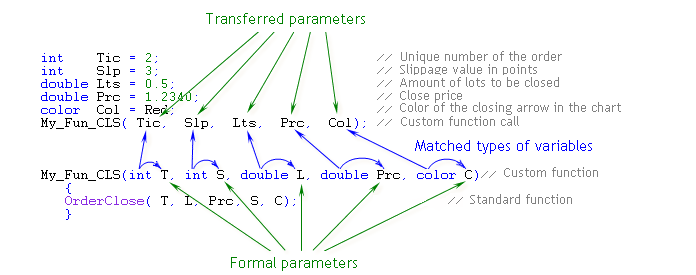Figure 22 The number, types, and order of passed and formal parameters match. Only variables are used as passed parameters.Only variables, expressions, and constants can be used as passed parameters.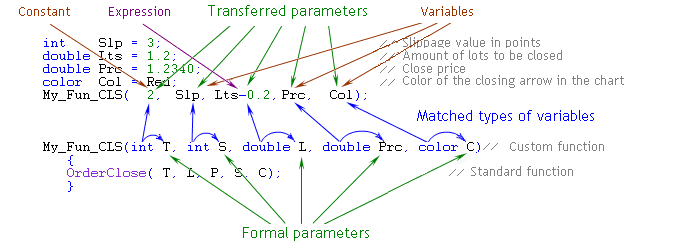Figure 23 The number, types, and order of passed and formal parameters match. A constant, an expression, and variables of the corresponding type are used as passed parameters.

## Formal parameters

A major feature of functions is the use of formal parameters.

Formal parameters are a list of variables specified in the header of the function description.

We mentioned before that one function can be used in several programs. However, different programs may use different names for the variables. If functions required strict matching in the names of variables (and, correspondingly, in their values), it would not be convenient for programmers. Indeed, you would then have to compose each newly developed program in the names of variables that had already been used in your functions before. However, fortunately, the variables used inside functions are not related to the variables used in a program.

Let us refer to Figures 20 and 21 once again. It should be noted that the names of the passed parameters (A and B, given in the parentheses of the function call) do not match with the names of the parameters (a and b) specified in the function description. We noticed in the Constants and variables that MQL4 is case-sensitive. Thus, the names A and a, and B and b considered here are different names of variables. However, there is no error in this code.

The variables (formal parameters) used in the function description are not related to the variables used in the basic code of the program. They are just different variables. Only variables, not constants, can be specified in the function header as formal parameters.

## How it works

In the program, there occurs a function call, variables A and B being specified in the parentheses of the call.

The program calls the function of the same name, which has formal parameters a and b specified in its header.

The value of variable A is assigned to variable a.

The value of variable B is assigned to variable b.

The executable function performs calculations using the values of variables a and b.

You may use any name for a formal parameter, but the name must not coincide with any of the names of the variables used in the program. In this example, we used the identifiers of formal parameters a and b. However, we could use any others, for example, m and n, or Kat_1 and Kat_2. Of course, when writing a program, you should specify in the function body the calculations which use the names of variables given in the header. Since we have given a and b in the header, so we have to use in the function a and b, not m and n.

In a function, the calculations are made that involve formal parameters a and b, but not variables A and B. In a function, any allowed actions may be perform on formal parameters a and b, including changes in the values of these variables. This does not have any effect on variables A and B.

The return value calculated in the function is given in the parentheses of the operator return(). The value of a variable, the result of an expression, or a constant can be used as return value. In our case, this is the value of local variable c. (A local variable is a variable declared within a function; if you exit the function, the values of all local variables will be lost; we will consider local variables in detail in Types of variables.) The function returns to the program the value of local variable c (as in Figure 19). This means that this value will now be assigned to variable C.

Further calculations in the program, if any, can be performed with the variables declared within the calling function. In our case, the calling function is special start() function, which contains the line for calling the user-defined function, whereas the variables declared within the calling function are A, B, and C. Thus, in the function, the calculations are performed using formal parameters, which allows us to create functions using arbitrary names of variables, irrespective of the names of values actually used in the program.

## Example: standard functions in a program

First of all, let us consider the behavior of the program shown in Figure 18. We should note that the entire code of the program is located inside the special start() function. At this training phase, we will not pay special attention to this. (Special functions and their properties are considered in detail in Special functions.)

Let us follow the execution of the program starting with the assignment operator.

` int A = 3;                               // First cathetus`

1. The right part of the assignment operator contains the specified constant; its value is three.

2. The value of three (the value of the right part) is assigned to variable A (located to the left of the equality sign in the assignment operator).

The control is given to the next line.

`   int B = 4;                               // Second cathetus`

3. The right part of the assignment operator contains the specified constant; its value is four.

4. The value of four is assigned to variable B.

The program goes to execution of the next line.

`  int C_2 = A*A + B*B;                      // Sum of the squares of catheti`

5. This execution starts with the calculation of the right part of the assignment operator. The result of the calculations is the value of 25. (The details of how a program refers to variables to take their values are considered in Constants and variables.)

6. Assignment of the value 25 to variable C_2.

The next line represents an assignment operator, the right part of which contains a call for the standard function.

`   int C = MathSqrt( C_2);                  // Calculation of hypotenuse`

The program seeks to execute the assignment operator. For this purpose, it executes calculations to the right of the equality sign first.

7. The program calls for execution of the standard function MathSqrt(), which calculates square roots. The value of variable C_2, 25, will be the passed parameter. Note that there is no description of this standard function anywhere in the program. The descriptions of standard functions must not be located in programs. (In the text of a program, you can easily distinguish the standard function call by its appearance; they are highlighted in MetaEditor with dark blue by default, but you may choose a different color.)

8. The calculations are made in the standard function MathSqrt().

9. The standard function MathSqrt() has completed its calculations. It returns the obtained value, five (the square root of 25).

The value returned by the function is now the content of the record.

`           MathSqrt( C_2)`

This record can be considered as a special, complex variable, a thing inside which the calculations are performed. After these calculations have been completed, the thing takes a value. The value returned by the function can be assigned to another variable or considered somehow in any other calculation.

10. In this case, our value is the value of the right part of the assignment operator. On continuing execution of the assignment operator, the program will assign the value of five to variable C.

11. The next line contains an operator that calls the standard Alert() function.

`   Alert("Hypotenuse = ", C);                // Message on the screen`

The standard Alert() function opens a dialog box where the values of the passed parameters are displayed. In this case, the function has taken two values as the passed parameters: a string constant "Hypotenuse = ", and an integer variable C which is five.

It was noted above that not all functions must return a value. The standard Alert() function does not return any value, since it has another taskit must display the text on the screen in a special window.

As a result of execution of the standard Alert() function, a window will appear on the screen with the following line:

Hypotenuse = 5

12. The last operator in this program completes the work of the special start() function.

` return;                                     // Function exit operator`

The work of the program is finished at this point.

A question may arise: how can we know which standard function will return a value, and which will not? The answer to this question is obvious: in order to find the detailed descriptions of built-in functions, you should read the reference Documentation in MQL4.community, the website launched by MetaQuotes Ltd., or the Help File of MetaEditor. The properties of a user-defined function are specified in its description. Whether a user-defined function returns a value or not depends on its algorithm; you decide while writing the program code of the function.

## Example: user-defined function in a program

Let us consider how the same calculations are made in the program containing a user-defined function in Figure 19. A certain part of the code that could previously be found in the special start() function is unavailable now. It is replaced with the call for the user-defined function. However, the special start() function is followed by the description of the user-defined function.

The first two lines, in which integer variables A and B take numeric values, remain the same. Consequently, nothing changes in their execution.

```   int A = 3;                            // First cathetus
int B = 4;                            // Second cathetus```

In the third line we have the assignment operator. Its right part contains the call for the user-defined function.

`   int C = Gipo(A,B);                    // Calculation of hypotenuse`

6. On executing this operator, the program will first call the user-defined function.

Note that the description of the user-defined function must be present in your program and placed immediately after the brace that closes the special start() function (that is, outside the special function).

In referring to the user-defined function, the program will perform the following steps.

6.1. Calling for variable A in order to get its value (in our case, three)

6.2. Calling for variable B in order to get its value (in our case, four)

Note that as soon as the program starts to call the user-defined function (the function is user-defined, in our specific case, but this rule applies to all functions), the program gets just a copy of the values of variables used as passed parameters, whereas the values of these variables themselves (in this case, A and B) are not supposed to change due to application of the user-defined function, nor do they really change.

7. The control is passed to the user-defined function.

During the whole time of execution of the user-defined function (no matter how long it takes), the values of variables in the calling program will not get lost, but will be stored.

The first line in the description of the user-defined function is its header.

`   int Gipo(int a, int b)                 // User-defined function`

On executing the user-defined function, the program takes the following steps.

7.1. The value of three (the first value in the list of passed parameters) is assigned to variable a (the first variable in the list of formal parameters).

7.2. The value of four (the second value in the list of passed parameters) is assigned to variable b (the second variable in the list of formal parameters).

Then the control is passed to the function body for it to execute its algorithm.

The first operator in the function body is the following line.

`   int c2 = a*a + b*b;                   // Sum of the squares of catheti`

7.3. On executing this operator, the program calculates the value in the right part of the assignment operator, and then it assigns the obtained value (in our case, 3*3 + 4*4 = 25) to variable c2.

The next operator is the following line of code.

`   int c = MathSqrt(c2);                 // Hypotenuse`

7.4. Here we find the square root of the value of variable c2. The order of operations is the same as in the previous example. The description of the standard function is not used either. The execution of the assignment operator results in assigning the value of five to variable c.

7.5. In the next line, we have the following operator.

`   return(c);                           // Function exit operator`

On executing this operator, the program will return (to the call site of the user-defined function) the value enclosed in the parentheses of this operator. In our case, it is the value of variable c, five.

At this point, the execution of the user-defined function is over, the control is given back to the call site.

8. Recall that the user-defined function is called from the following operator.

`   int C = Gipo(A,B);                   // Hypotenuse calculation`

The record (the user-defined function call), at the stage of returning the value, takes the value calculated in the function, five.

`          Gipo(A,B)`

On completing the execution of the assignment operator, the program assigns the value of five to the variable C.

9. The next operator is executed in the same way as in the previous example.

`   Alert("Hypotenuse = ", C);           // Message on the screen,`

Namely, in a special window, a message appears:

Hypotenuse = 5

10. The last operator in this program completes the work of the special start() function, and it simultaneously completes the work of the entire program. (The properties of special functions are considered in detail in Special functions.)

` return;                                // Function exit operator,`

Let us consider some implementation versions of the above user-defined function. It is easy to verify that programming with user-defined functions has some incontestable advantages.

## Previously considered implementation of user-defined function Gipo()

In this function, the formal parameters "resemble" the variables used in the basic program. However, this is just a formal similarity, because A and a are different names of variables.

```//-----------------------------------------------------------------------
int Gipo(int a, int b)                  // User-defined function
{
int c2 = a*a + b*b;                  // Sum of the squares of catheti
int c = MathSqrt(c2);                // Hypotenuse
return(c);                           // Function exit operator
}
//-----------------------------------------------------------------------```

## Implementation of user-defined function Gipo(): version 2

In this case, the names of formal parameters do not "resemble" the names of variables used in the basic program. However, this does not prevent us from using this function in the program.

```//--------------------------------------------------------------------
int Gipo(int alpha, int betta)           // User-defined function
{
int SQRT = alpha*alpha + betta*betta; // Sum of the squares of catheti
int GIP = MathSqrt(SQRT);             // Hypotenuse
return(GIP);                          // Function exit operator
}
//--------------------------------------------------------------------```

## Implementation of user-defined function Gipo(): version 3

In this example, the variable alpha is reused in the program and changes its value twice. This fact has no effect on the actual variables specified in the function calls of the main program.

```//--------------------------------------------------------------------
int Gipo(int alpha, int betta)           // User-defined function
{
alpha= alpha*alpha + betta*betta;     // Sum of the squares of catheti
alpha= MathSqrt(alpha);               // Hypotenuse
return(alpha);                        // Function exit operator
}
//--------------------------------------------------------------------```

## Implementation of user-defined function Gipo(): version 4

In this user-defined function, all calculations are collected in one operator. The return value is calculated directly in the parentheses of the operator return(). The subduplicate is calculated directly in the location where the passed parameter must be specified in the standard function MathSqrt().This solution may seem to be strange or wrong at the beginning. However, there is no error in this implementation of the user-defined function. The number of operators used in the function is smaller than in other implementations, so the code is more compact.

```//--------------------------------------------------------------------
int Gipo(int a, int b)                   // User-defined function
{
return(MathSqrt(a*a + b*b));          // Function exit operator
}
//--------------------------------------------------------------------```

Thus, the application of user-defined functions has some incontestable advantages in the programming practice:

The names of variables in the basic text of the program have no relations to the names of formal parameters in a user-defined function.

User-defined functions can be reused in different programs; there is no need to change the code of a user-defined function.

Libraries can be created if necessary.

These very useful properties of functions allow you to create really large programs in a corporation, for example. Several programmers can be involved in this process simultaneously, and they are released from the necessity to agree about the names of variables they use. The only thing to be agreed is the order of variables in the header and in the function call.OperatorsProgram Types